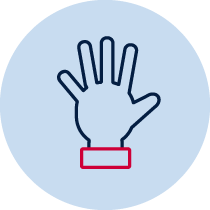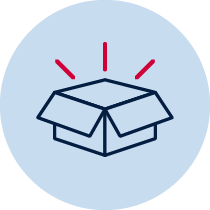# Mass – balance itPractical Resource required Whole class

## Measurement – mass

A student:

• measures, records, compares and estimates the masses of objects using uniform informal units MA1-12MG
• describes mathematical situations and methods using everyday and some mathematical language, actions, materials, diagrams and symbols MA1-1WM
• uses objects, diagrams and technology to explore mathematical problems MA1-2WM

## Syllabus content descriptors

• Investigate mass using a pan balance
• Compare the masses of objects using balance scales (ACMMG038)

• uses a range of strategies and informal recording methods for addition and subtraction involving one- and two-digit numbers.MA1-5NA
• Represent and solve simple addition and subtraction problems using a range of strategies, including counting on, partitioning and rearranging parts (ACMNA015)

## National Numeracy Learning Progression mapping to the NSW mathematics syllabus

When working towards the outcome MA1‑12MG the sub-elements (and levels) of Understanding units of measurement (UuM1-UuM6) describe observable behaviours that can aid teachers in making evidence-based decisions about student development and future learning.

## Materials

• pan/equal arm balance
• small items, for example, a pair of scissors, glue stick, stapler, toy
• uniform informal units for measuring for example counters, Unifix cubes, multi-attribute blocks (MAB) blocks

## Teacher instructions

The purpose of this task is to gauge students’ understanding of initial mass concepts such as:

• the terms ‘light’ and ‘heavy’
• comparing the mass of items using informal units
• estimating the mass of items
• using a pan or equal arm balance to compare mass.

Students will experiment with materials to draw conclusions. Teachers need to decide on the materials that will support students to access this task.

To complete questions 1 and 2, student success is dependent on their understanding of the terms ‘lighter’, ‘heavier’ and ‘about the same’ in reference to mass (from Early Stage 1 content). Teachers may need to explain this mathematical language to students.

It may be useful when students are completing question 4a, for the teacher to ask probing questions to gain more information about student understanding. Examples of probing questions include:

What did you already know that helped you come up with that estimate?

In question 3c – the term ‘difference’ may need to be explained to students prior to the completion of the task.

## Student instructions

Question 1

Look at the picture, 'I wonder which side you think is heavier and which one is lighter?'

a.) Can you draw items on the scale to show what you mean?

b.) Label each side ‘lighter’ and ‘heavier’ to match your picture.

Question 2

a) Use a pan balance to find two collections of items that have the same mass.

b) Draw, take a photo or video of your pan balance.

c) Describe your pan balance, for example record amounts of items in the collections

Question 3

a.) Choose an item to measure. How many of the same informal units does it take to balance this in the pan balance? Draw what it looks like and write your answer in the box.

b.) Choose another item to measure, and using the same informal units, estimate how many units it will take to balance this item in the pan balance. Record your estimate. How many of the same informal units did it take to balance this item in the pan balance? Draw what it looks like and write your answer in the box.

c.) What is the difference in mass between the two items you measured?

## Possible areas for further exploration?

Question 3 – When discussing this task, students may indicate that they knew the second item was lighter/heavier than the first item and therefore would need less/more units to balance it. This response would indicate that the student is using the first item as a benchmark to compare further items against. They are then working towards being able to transfer this knowledge to formal units of measurement.

If a student were to respond with ‘I guessed’, this would indicate that they may not understand the term ‘estimate’ and this may need to be explained further. It may also indicate that the student may be able to measure an item accurately using equipment but that they don’t yet have an understanding of how the concept of mass is applied in real-life situations.

## Where to next?

If students are confident with the requirements of this task, teachers may encourage further investigation of the ‘difference’ of the mass of two items.

Discuss why some informal units are not uniform (such as pebbles) and investigate how this affects when measuring mass.

Creating or solving word problems related to mass.

Syllabus outcomes and content descriptors from Mathematics K-10 Syllabus © NSW Education Standards Authority (NESA) for and on behalf of the Crown in right of the State of New South Wales, 2012